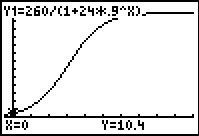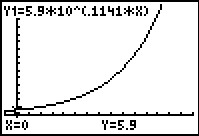••• ##### Device
• TI-83 Plus Family
• TI-84 Plus
• TI-84 Plus Silver Edition
•TI-84 Plus C Silver Edition
•TI-84 Plus CE
• ##### Report an Issue

Precalculus: Domain & Range
by Texas Instruments - Bell Ringer Lesson#### Overview

Students explore a variety of mathematical functions and real world applications of functions to understand the concepts of domain and range.

#### Key Steps

•In this activity, students find the domain and range of various real-world problems. The first problem involves exploring an equation that models the height of a sunflower plant over time.

•There are extra problems at the end of the activity for students to complete either in class or for homework. These problems include sine, logarithmic, quadratic, and square root functions.